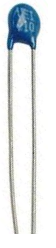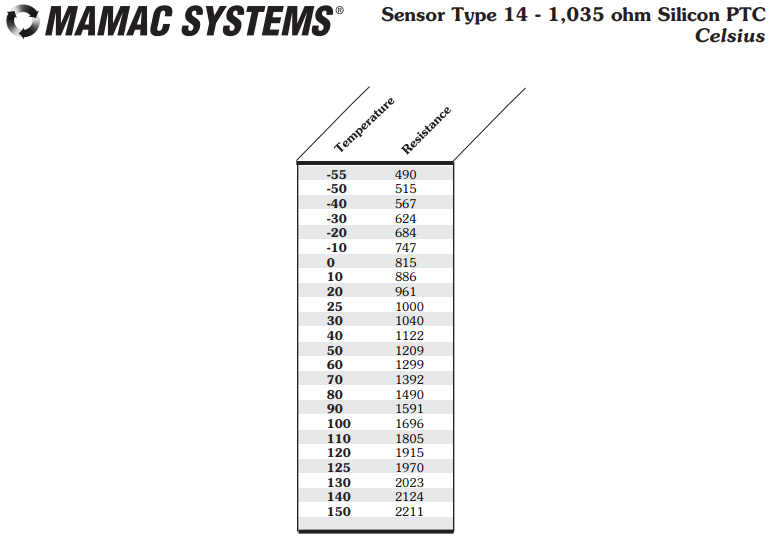﻿ How to Use a Thermistor Output Table ﻿# How to Use a Thermistor Output TableIn this article, we show how to use a thermistor output table.

When designing circuits with thermistors, it is important many times to know what resistance the thermistor will output at a certain given temperature.

This way, you can calculate the voltage output for any given temperature that the thermistor output table covers.

A thermistor is a variable resistor whose resistance varies in proportion to the temperature that the thermistor is exposed to.

There are 2 types of thermistors- NTC and PTC. The resistance of NTC thermistors vary inversely with temperature. The resistance of PTC thermistors vary directly with temperature.

NTC thermistors are the most commonly used type of thermistor, and NTC thermistors are used in several different types of applications such as ovens and fire detectors. PTC thermistors are less common but are used in applications such as equipment with a near-zero reset time, extreme temperature conditions, and systems that experience frequent shorts.

Thermistors are nominally rated according to their resistance at a certain given temperature such as near room temperature such as 25°C.

Usually thermistors are at their rated resistance at 25°C, which is more or less around room temperature.

So at room temperature, a 100KΩ NTC thermistor will output a resistance of 100KΩ.

NTC thermistors have a resistance inversely proportional to temperature. If the temperature is low, NTC thermistors offer a very high resistance. If the temperature is high, NTC thermistors have a very low resistance.

The datasheet for an NTC thermistor should have a Thermistor Output Table, which tells us the resistance that the thermistor will output at various given temperatures.

This is shown in the table below.The complete datasheet for a 100K thermistor is shown in the following link: 100K Thermistor Datasheet.

You can see based on the thermistor output table that the temperatures that are covered range from -39°F or -39.44°C in the cold region to 187°F or 86.11°C in the hot region. For the most part, that will cover all the temperature ranges that the thermistor should be exposed to.

So based on these resistor outputs, we can use ohm's law to calculate the voltage output, which can then be read by microcontroller or ADC device, which can interpret the voltage as a temperature reading.

One of the most common ways of using a thermistor in a temperature sensor circuit is putting the thermistor in a voltage divider circuit with a fixed resistor. The fixed resistor will always have the same resistance output regardless of temperature with only possible minor fluctuations. On the other hand, the thermistor's resistance will fluctuate greatly with temperature, with an NTC thermistor having very high resistance at cold temperatures and low resistance at high temperatures.

You can see at the coldest extreme at -39°F or -39.44°C that the NTC thermistor outputs a resistance of 3916295Ω. At the total other extreme at the hottest temperature at 187°F or 86.11°C, the thermistor outputs a resistance of 8783Ω.

So during extremely cold conditions, in a voltage divider circuit with a fixed resistor of 100K, most of the supply voltage falls across the thermistor.

Doing the math, at -39°F or -39.44°C, 4.875V (5V(3.9M)/(3.9M + 100K)) would be dropped across the thermistor of the supplied 5V.

During extremely hot conditions, in the voltage divider circuit with a 100KΩ resistor, most of the supply voltage falls across the 100KΩ fixed resistor.

Doing the math, at 187°F or 86.11°C, 0.37V (5V(8K)/(8K + 100K)) would be dropped across the thermistor of the supplied 5V.

This was an example of an NTC nonlinear thermistor output table.

Just to show another example of a themistor, below we show a PTC thermistor output table.This is a 1035Ω PTC thermistor.

The temperature shown is in unit celsius.

You can see that it is the opposite than NTC thermistors, meaning that the output resistance has a direct relationship with the temperature. As the temperature increases, the output resistance increases. As the temperature decreases, the resistance decreases.

So being able to use a thermistor output table is important when you need to do precise calculations and read and interpret voltages output from a thermistor circuit. The thermistor is normally placed in a voltage divider circuit with a fixed resistor. The thermistor's varying resistance will change the output voltage. Based on calculations, you can know the temperature based on the voltage output from the circuit.

And this is how to use a thermistor output table for a NTC or PTC thermistor circuit.

Related Resources